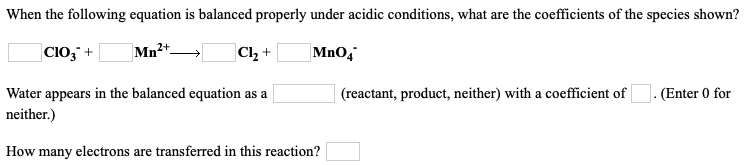# When the following equation is balanced properly under acidic conditions, what are the coefficients of the species shown? __ ClO3^- + ___ Mn^2+ → ___ Cl2 + ___ MnO4^- Water appears in the balanced equation as a ____ (reactant, product, neither) with a coefficient of ___. Enter 0 for neither.) How many electrons are transferred in this reaction?